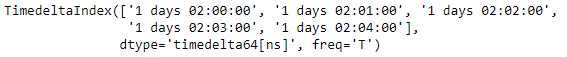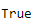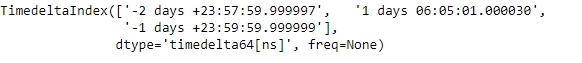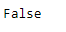# Python | Pandas TimedeltaIndex.is_monotonic

Python is a great language for doing data analysis, primarily because of the fantastic ecosystem of data-centric python packages. Pandas is one of those packages and makes importing and analyzing data much easier.

Pandas` TimedeltaIndex.is_monotonic` attribute checks if the given TimedeltaIndex object is monotonic or not. If the object is monotonic then the function return `True` else it return `False`. Monotonic means the values in object is varying in such a way that it either never decreases or never increases.

Syntax: TimedeltaIndex.is_monotonic

Return : boolean

Example #1: Use `TimedeltaIndex.is_monotonic` attribute to check if the TimedeltaIndex object is monotonic or not.

 `# importing pandas as pd ` `import` `pandas as pd ` ` `  `# Create the TimedeltaIndex object ` `tidx ``=` `pd.TimedeltaIndex(start ``=``'1 days 02:00:00'``, periods ``=` `5``, freq ``=``'T'``) ` ` `  `# Print the TimedeltaIndex ` `print``(tidx) `

Output :Now we will check if the TimedeltaIndex object is monotonic or not.

 `# check if tidx is monotonic or not ` `tidx.is_monotonic `

Output :As we can see in the output, the `TimedeltaIndex.is_monotonic` attribute has returned `True` indicating that tidx is monotonic.

Example #2: Use `TimedeltaIndex.is_monotonic` attribute to check if the TimedeltaIndex object is monotonic or not.

 `# importing pandas as pd ` `import` `pandas as pd ` ` `  `# Create the TimedeltaIndex object ` `tidx ``=` `pd.TimedeltaIndex(data ``=``[``'-1 days 2 min 3us'``, ``'1 days 06:05:01.000030'``, ` `                                                 ``'-1 days + 23:59:59.999999'``]) ` ` `  `# Print the TimedeltaIndex ` `print``(tidx) `

Output :Now we will check if the TimedeltaIndex object is monotonic or not.

 `# check if tidx is monotonic or not ` `tidx.is_monotonic `

Output :As we can see in the output, the `TimedeltaIndex.is_monotonic` attribute has returned `False` indicating that tidx is not monotonic.

My Personal Notes arrow_drop_upCheck out this Author's contributed articles.

If you like GeeksforGeeks and would like to contribute, you can also write an article using contribute.geeksforgeeks.org or mail your article to contribute@geeksforgeeks.org. See your article appearing on the GeeksforGeeks main page and help other Geeks.

Please Improve this article if you find anything incorrect by clicking on the "Improve Article" button below.

Article Tags :

Be the First to upvote.

Please write to us at contribute@geeksforgeeks.org to report any issue with the above content.